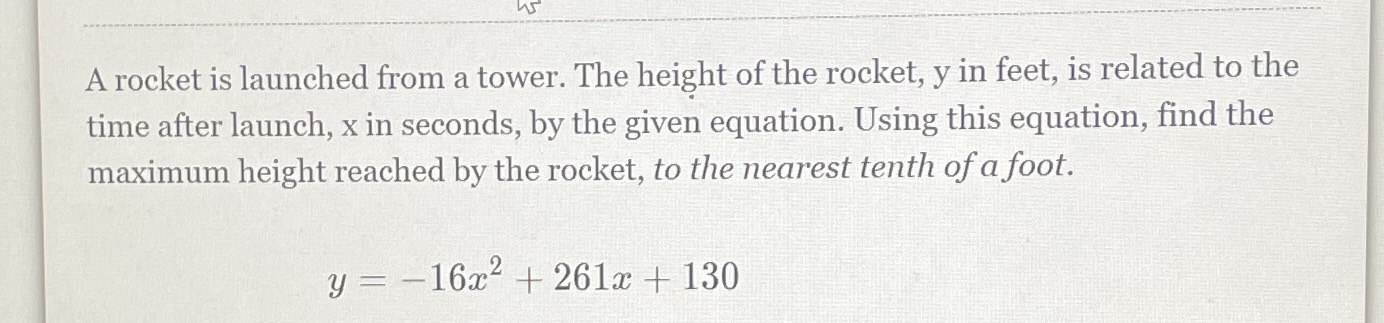Still have math questions?

Geometry
QuestionA rocket is launched from a tower. The height of the rocket,y in feet,is related to the time after launch,x in seconds,by the given equation. Using this equation,find the maximum height reached by the rocket,to the nearest tenth of foot.

$$y=-16x^{2} +261x+130$$

$$\frac{76441}{64}$$